# Basic Hydraulics - Fluid Power Physics

### CHAPTER 1 - Fluid Power Physics

ENERGY
As we begin our study of basic hydraulics we must first recognize that fluid power is another method of transferring energy. This energy transfer is from a prime mover or input power source, to an actuator or output device. This means of energy transfer, although not always the most efficient, where properly applied may provide optimum work control. Energy is defined as the ability to do work.

WORK
Work is defined as force through a distance. If 1000 pounds is moved a distance of 2 feet, work has been accomplished. Work is measured in foot-pounds.

Work (in • lbs) = Force (lbs) x Distance (in)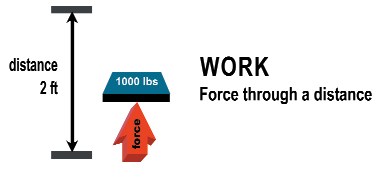Figure 1.1 –illustration of work
• Example 1.1 - In Figure 1.1, 1000 pounds is moved a distance of 2 feet. After the weight is moved a distance of two feet, how much work has been accomplished?
• Solution: Work (in.lbs) = 1000 (lbs) x 2ft = 2000 ft.lbs
• Answer: 2000 ft.lbs of work

POWER
Power is defined as the rate of doing work, or work over time. In order to have relative meaning for measuring power, power must be converted to horsepower, which is a unit of measuring energy

Power = Force x Distance/ Time or Work/ Time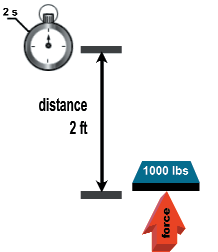Figure 1.2 - illustration of power
• Example 1.2 - In Figure 1.2, 1000 pounds is lifted a distance of two feet in two seconds. How much power has been accomplished?
• Solution: Power = 1000 (lbs) x 2 ft/ 2 s = 1000 lb.ft/s

HORSEPOWER
Mathematically, hydraulic horsepower is expressed as follows: horsepower equals flow, in gallons per minute (gpm), times pressure, inch-pounds per square inch (psi), divided by 1714, a constant.

Hydraulic HP = Flow (gpm) x Pressure (psi)/ 1714 (constant)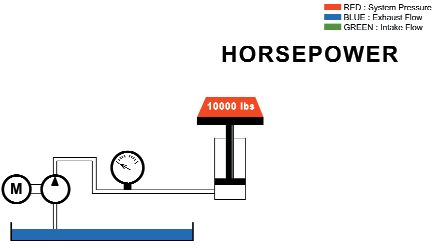Figure 1.3 - illustration of hydraulic horsepower
In Figure 1. 3 , a 10,000 pound weight is being lifted a distance of 1 foot. If the load is lifted in 2 seconds, a power requirement is defined. This may be expressed as hydraulic horsepower. To lift 10,000 pounds a distance of 1 foot in 2 seconds, there must be a required flow rate at a specific pressure, based on cylinder size and the pump flow discharge.
• Example 1.3 - In Figure 1.3, the 10,000 pound weight is lifted a distance of 1 foot in 2 seconds. If the pump is providing 15 gpm and the pressure requirement to lift the 10,000 pounds is 1700 psi, what is the theoretical horsepower requirement?
• Solution: Hydraulic HP = 15 (gpm) x 1700 (psi)/ 1714 (constant) = 14.88 HP

HEAT
The law of conservation of energy states that energy can neither be created nor destroyed, although it can change its form. Energy in a hydraulic system that is not used for work takes the form of heat.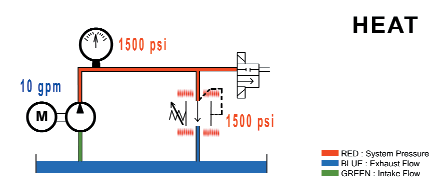Figure 1.4 - wasted horsepower in a hydraulic system is converted into heat
• Example 1.4 - In Figure 1.4, 10 gpm are going through a relief valve, which has a pressure setting of 1500 psi. What is the amount of energy being converted to heat?
• Solution: Hydraulic HP = 10 (gpm) x 1500 (psi)/ 1714 (constant) = 8.75 HP

TORQUE
Torque is twisting force. Mathematically, torque is defined as force times a moment arm and is
measured in foot-pounds. The moment arm is the distance from the object on which the force is being applied to the actual force itself.

Torque = Force x Moment Arm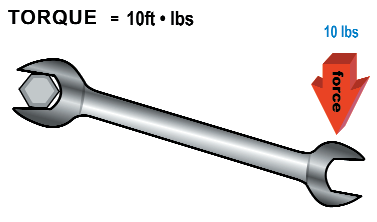Figure 1.5 - illustration of torque
This same theory applies to hydraulic motors. Hydraulic motors are actuators that are rated in specific torque values at a given pressure. The twisting force, or torque, is the generated work. A motor’s rotation per minute (rpm) at a given torque specifies our energy usage or horsepower requirement.
• Example 1.5 - In Figure 1.5, 10 lbs of force is being applied to a wrench 1 foot in length. What is the amount of torque being produced on the bolt?
• Solution: 10 lbs is the force. The moment arm in this example is the distance between the force and the bolt. Therefore, we have a moment arm of 1 foot. Torque = 10 lbs x 1 ft = 10 ft.lbs
• Answer: 10 ft.lbs of torque

FLOW
Flow in a hydraulic system is produced from a positive displacement pump. This is different from a centrifugal pump, which is not positive displacement. There are three important principles that must be understood relating to flow in a hydraulic system.
• Principle one: Flow makes it go. For anything to move in a hydraulic system the actuator must be supplied with flow. In Figure 1.6, when the cylinder is retracted, it can extend only if there is flow into port B. Shifting the directional control valve will send flow to either extend or retract the cylinder.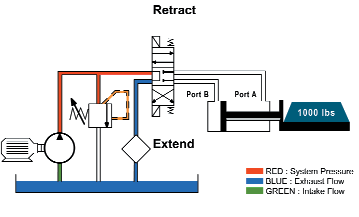Figure 1.6 - a simple hydraulic system
• Principle two: Rate of flow determines speed. Rate of flow is usually measured in gallons per minute (gpm). The pump determines gpm. Changes in pump output flow will change the speed of the actuator.
• Principle three: With a given flow rate, changes in actuator volume displacement will change actuator speed. With less volume to displace, the actuator will cycle faster. For example, referring to Figure 1.6 again, there is less volume to displace when the cylinder is retracted, because the cylinder rod occupies space, thus diminishing the volume to be displaced.

PRESSURE
Pressure in a hydraulic system comes from resistance to flow. To further illustrate this principle, consider the flow produced from the hydraulic pump in Figure 1.7. The pump is producing flow, not pressure. However, as flow from the pump is restricted, pressure will result. This resistance to flow is load induced from the actuator and also generated as the fluid is passed through the various conductors and components. All points of resistance, such as long runs of pipe, elbows, and various components, are accumulative in series and contribute to total system pressure.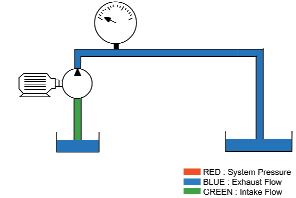Figure 1.7 - the hydraulic pump is not producing pressure
Pascal’s law forms the basis for understanding the relationship between force, pressure, and area. The relationship is often expressed with the symbol in Figure 1.8.Figure 1.8 - Pascal's law triangle
Mathematically we express this relationship as: Force is equal to pressure times area; pressure is equal to force divided by area; and area can be calculated by dividing force by pressure.

Force = Pressure x Area
Pressure = Force/ Area
Area = Force/ Pressure

Pascal’s law is expressed as follows: Pressure applied on a confined fluid at rest is transmitted undiminished in all directions and acts with equal force on equal areas and at right angles to them.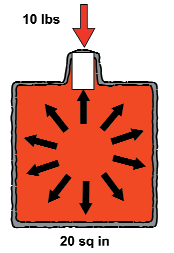Figure 1.9 - Pascal’s law applied to a container of fluid
• Example 1.6 - In Figure 1.9, a vessel is filled with a non-compressible liquid. If 10 pounds of force is applied to the 1 square inch stopper, and the bottom of the container is 20 square inches, what is the result, in force, on the bottom of the container?
• Solution: Pascal’s law states that pressure applied on a confined fluid at rest is transmitted undiminished in all directions and acts with equal force on equal areas and at right angles to them. Therefore, 10 pounds of force would result on every square inch of the container wall. Force = 10 psi x 20 in2 = 200 lbs
• Answer: 200 lbs of force

Load-induced pressure is defined as pressure generated from the load, or force on the actuator. The effective area of the cylinder piston is the area available for force generation. In Figure 1.10, a 10,000 pound force gives a load-induced pressure of 1,000 psi, based on the formula. When the cylinder is extended, the required pressure to move the 10,000 pound load is 1,000 psi less frictional forces.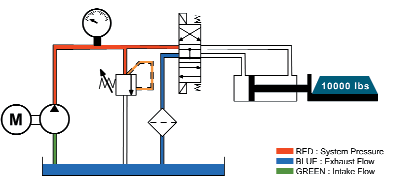Figure 1.10 - pressure is induced by the load on the cylinder
During retraction, the effective area is only 5 square inches. This increases the required pressure to 2,000 psi needed to retract the load.

PRESSURE DROP
Pressure that is not directly used to provide work may be defined as pressure drop or resistive pressure. It is the pressure required to push the fluid through the conductors to the actuator. This energy takes the form of heat. Excessive pressure drop may contribute to excessive heat build up in the hydraulic system. This resistive pressure is accumulative and must be added to the overall system pressure requirements.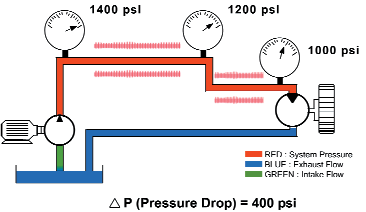Figure 1.11 - pressure drop may result in heat build up

FLUIDS
The study of fluid power deals with understanding energy transmission through a confined liquid. The hydraulic fluid may well be considered the most important component in a hydraulic system. It serves as lubricant, heat transfer medium, a means of transferring energy, and a sealant.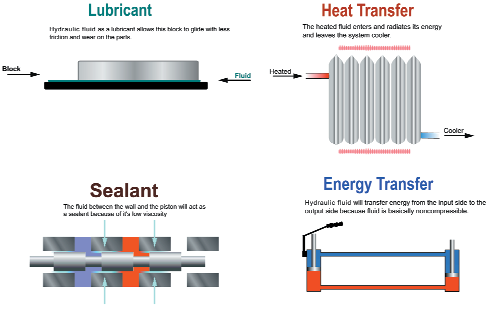Figure 1.12 - fluids in a hydraulic system serves multiple purposes
Hydraulic fluid is basically non-compressible and can take the shape of any container. Because of this, it exhibits a certain advantage in the transmission of force. Figure 1.13 is an example where the fluid will take the shape of a container. Using a positive displacement pump energy is transmitted from the prime mover (input source) to the actuator (output) through the medium of a non-compressible fluid. As the fluid passes through the conductors and components, certain considerations must be given to ensure maximum efficiency of energy transfer. These considerations include the understanding and proper application of fluid velocity and viscosity.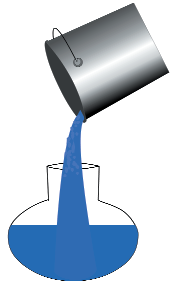Figure 1.13 - hydraulic fluid will take the shape of its container

VELOCITY
Velocity is the distance fluid travels per unit of time. With a fixed volume of fluid going through a
conductor, the velocity of the fluid will depend on the inside diameter of the conductor.

Velocity = (gpm) x 0.3208/ Inside Diameter x 0.7854

If the diameter of a conductor is increased, the velocity of the fluid will decrease. Conversely, if the diameter of the conductor is decreased, the fluid’s velocity will increase.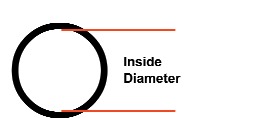Figure 1.14 - inside diameter of a fluid conductor
To better illustrate this principle two simple systems are shown in Figure 1.15, in which two pumps of equal 30-gpm displacement move fluid through conductors of different sizes. The displacement
remains equal while the velocity of the fluid varies with the size of the conductor. The fluid turning fly wheel 2 is moving 4 times faster than the fluid turning fly wheel 1 because the inside diameter of the pipe for fly wheel 1 is twice the size of the inside diameter of fly wheel 2. However, the flywheels turn at the same rate because the volume displacement is equal in both systems.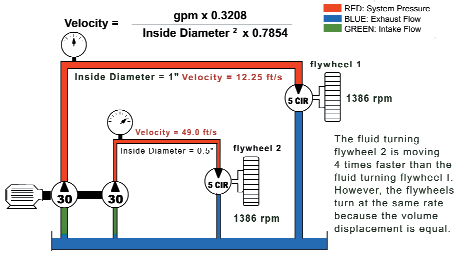Figure 1.15 - a simple hydraulic system

VISCOSITY
Viscosity is a measure of a liquid’s resistance to flow. A thicker fluid has more resistance to flow and a higher viscosity. Viscosity is affected by temperature. As a hydraulic fluid’s temperature increases, its viscosity or resistance to flow decreases.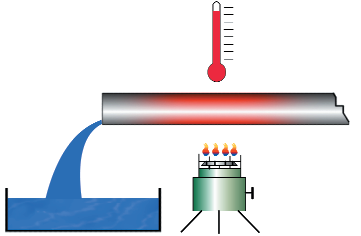Figure 1.16 - an example of a fluid’s viscosity
A viscometer (Figure 1.17), the device used to measure a liquid’s viscosity, consists of a small reservoir surrounded by a water bath used to heat and maintain the liquid at a constant temperature. There is a small orifice below the reservoir through which the liquid can pass once it is heated to a specified temperature. A stopwatch is used to determine how much time it takes to fill a 60-milliliter flask. The number of seconds that it takes to fill the flask at a given temperature is the liquid’s viscosity at that temperature.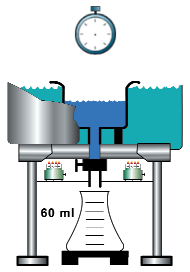Figure 1.17 - an example of a viscometer

Blog.Teknisi

### 3 Responses to "Basic Hydraulics - Fluid Power Physics"

1.This information is useful to us. You have shared such an useful article. I get an information for Magnetic Actuator by your blog .

2.Extremely useful information which you have shared here about Permanent magnets. This is a great way to enhance knowledge for us, and also helpful for us. Thankful to you for sharing an article like this.

3.You are giving such interesting information about Thermistors for sale online in USA. It is great and beneficial info for us, I really enjoyed reading it. Thankful to you for sharing an article like this.